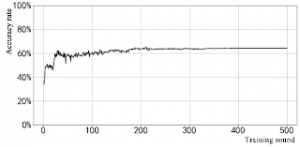## How to Calculate and Solve for Actual Mass of Geological Body | GravityThe image above represents actual mass of geological body.

To compute for actual mass of geological body three essential parameters are needed and these parameters are Total Anomalous Mass (ME), Anomalous Density of the Body (ρ1and Host Rock Density (ρ0).

The formula for calculating actual mass of geological body:

M = ME (ρ1 / ρ1 – ρ0)

Where:

M = Actual Mass of Geological Body
ME = Total Anomalous Mass
ρ1 = Anomalous Density of the Body
ρ0 = Host Rock Density

Let’s solve an example;
Find the actual mass of geological body when the total anomalous mass is 12, anomalous density of the body is 21 and the host rock density is 13.

This implies that;

ME = Total Anomalous Mass = 12
ρ1 = Anomalous Density of the Body = 21
ρ0 = Host Rock Density = 13

M = ME (ρ1 / ρ1 – ρ0)
M = 12 (21 / 21 – 13)
M = 12 (21 / 8)
M = 12 (2.625)
M = 31.5

Therefore, the actual mass of geological body is 31.5 kg.